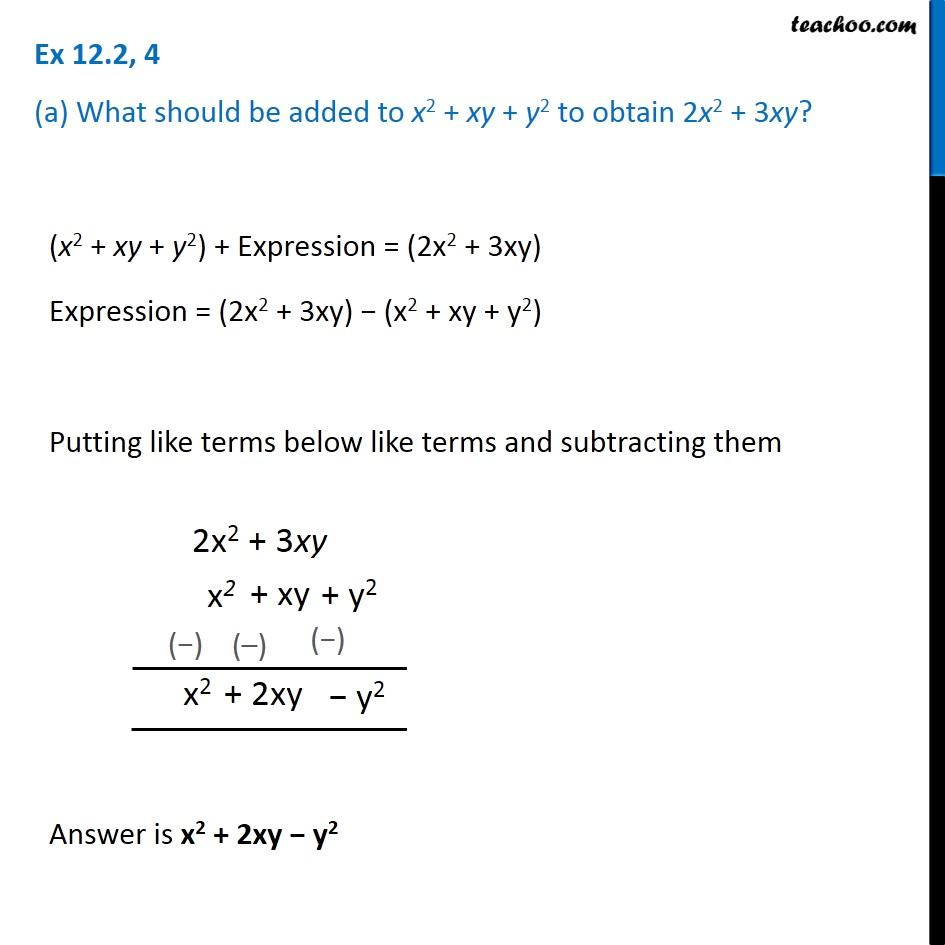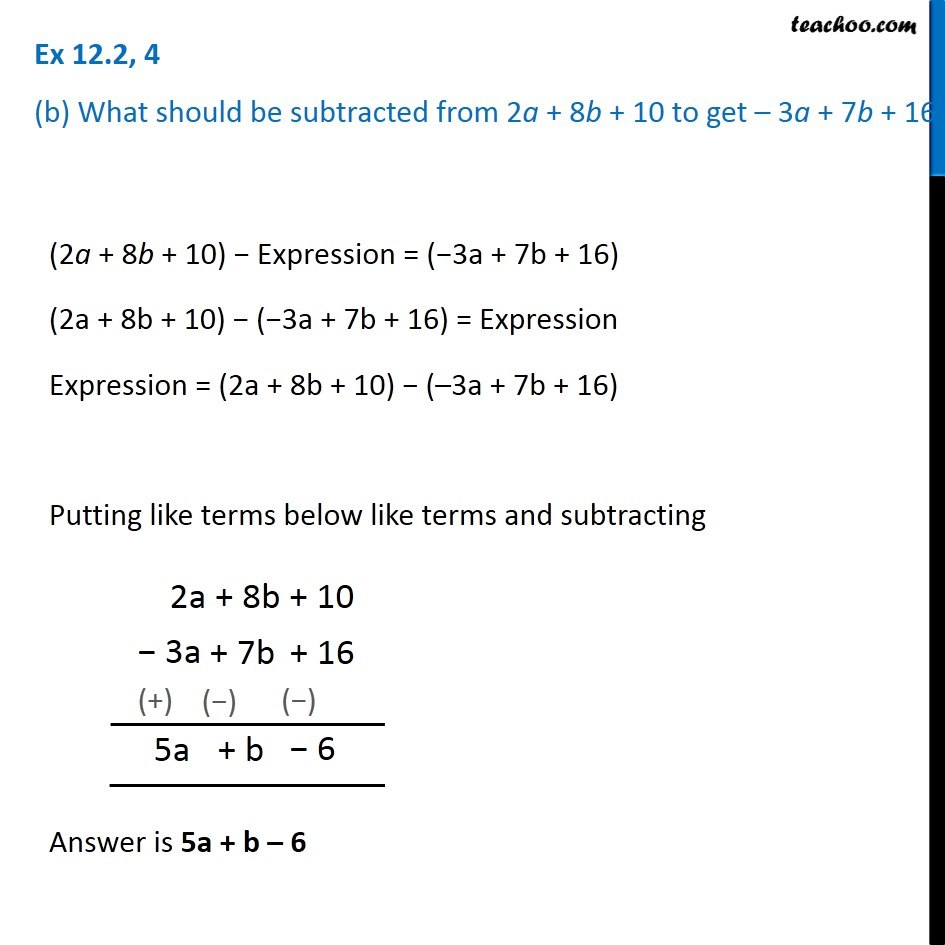Subscribe to our Youtube Channel - https://you.tube/teachoo

1. Chapter 12 Class 7 Algebraic Expressions
2. Serial order wise
3. Ex 12.2

Transcript

Ex 12.2, 4 (a) What should be added to x2 + xy + y2 to obtain 2x2 + 3xy? (x2 + xy + y2) + Expression = (2x2 + 3xy) Expression = (2x2 + 3xy) − (x2 + xy + y2) Putting like terms below like terms and subtracting them Answer is x2 + 2xy − y2 Ex 12.2, 4 (b) What should be subtracted from 2a + 8b + 10 to get – 3a + 7b + 16? (2a + 8b + 10) − Expression = (−3a + 7b + 16) (2a + 8b + 10) − (−3a + 7b + 16) = Expression Expression = (2a + 8b + 10) − (–3a + 7b + 16) Putting like terms below like terms and subtracting Answer is 5a + b – 6

Ex 12.2